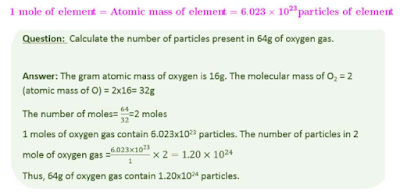# Atoms and Molecules Class 9 Notes Science Chapter 3

By -

Atoms and Molecules Class 9 Notes - We have provided a synopsis as well as revision notes for Class 9 Science Chapter 3. This set of CBSE notes includes CBSE Key Notes, CBSE Revision Notes, Short Key Notes, photos, and diagrams for the entire Chapter 3 named Atoms and Molecules of Science, which was taught in class 9. If you are a class 9 student utilising the NCERT Textbook to study Science, you will come across Chapter 3 Atoms and Molecules. You must be looking for notes to memorise after you have studied the lecture. Complete Chapter 3 Atoms and Molecules class 9 notes are available here. You should also look through NCERT Solutions for Class 9 Science subject 3 Atoms and Molecules for a better understanding of this subject.

## Atoms and Molecules

Laws of Chemical Combination

⇒ Antoine L. Lavoisier gave important laws of chemical combination which law the foundation of chemical science. There are two important laws of chemical combination:

(a) Law of conservation of mass:

The Law of conservation of mass states that mass can neither be created nor destroyed in a chemical reaction. In a chemical reaction,

Total Mass of the Reactants = Total Mass of the Products

(b) Law of constant proportions:

According to this law, the elements are always present in definite proportions by mass in a chemical substance. This law is also called the law of definite proportions.

Dalton's Atomic Theory:

• The matter is made up of tiny particles which are called an atom.

• The atoms can neither be created nor destroyed in a chemical reaction.

• The atoms of the given element have similar mass and chemical properties and vice versa.

• The compound can be formed when atoms of the same or different elements combine in a fixed ratio.

• The relative number and kinds of atoms are constant in a given compound.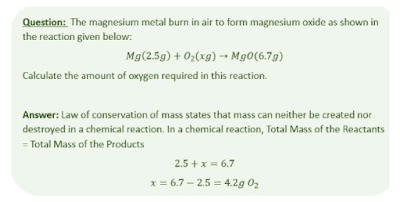What is an Atom?

⇒ The matter is made up of tiny particles which are called an atom.

⇒ Atoms are very small, they are smaller than anything that we can imagine or compare with. Atomic radius is measured in nanometers. (nm).

Chemical Symbol:

⇒ A chemical symbol is defined as the notation of one or two letters that represent a chemical element.

⇒ The chemical symbol of some elements is derived from the first letter of the name and a letter appearing later in the name of that element. For example, the symbol of carbon is C.

⇒ Some symbols are derived from the Latin name of that element. For example, the symbol of sodium is 'Na' which is derived from its Latin name, which is 'Natrium.'

The list of the chemical symbol of certain elements is shown below: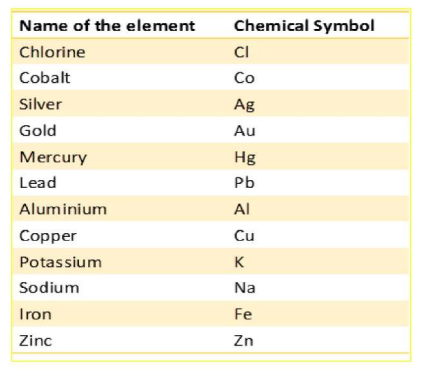Atomic mass:

⇒ The atomic mass of an element gives us the mass of one atom of that element in atomic mass units (u).

⇒ One atomic mass unit is equal to 1/12 the mass of one atom of carbon-12.

⇒ The chemical symbol and atomic mass of the given elements are shown below: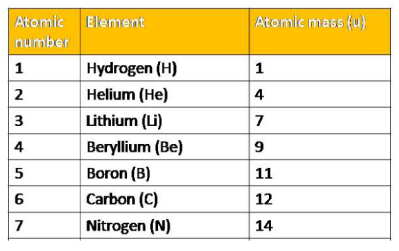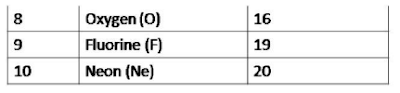What is a Molecule?

⇒ A molecule is, in general, a group of two or more atoms that are chemically bonded together, that is, tightly held together by attractive forces.

⇒ The number of atoms constituting a molecule is known as its atomicity.

⇒ The molecule that contains two atoms is diatomic while the triatomic molecule contains 3 atoms. The polyatomic molecule contains more than 3 atoms.

Molecule of element:

The molecule of an element contains two or more atoms of similar atoms.

⇒ A list of certain molecules is given below: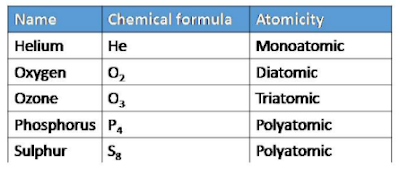Molecule of compound:

The molecule of a compound contains two or more atoms of different elements.

⇒ A list of certain molecules is given below: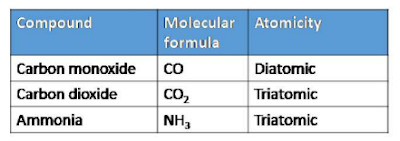⇒ The ionic compound contains positively charged ion (cation) and negatively charged ion (anion). For example, sodium chloride contains Na+ ion and Cl- ion. Sodium loses an electron to form Na+ ion while chlorine accepts an electron to form Cl- ion.

Writing Chemical Formulae

⇒ The chemical formula is the notation used to show the number and type of atom present in a molecule using chemical symbols and numerical subscripts.

⇒ The charged species is called an ion. The negatively charged ion is called anion while the positively charged ion is called a cation.

⇒ A group of atoms carrying a charge is known as a polyatomic ion. The chemical formula must contain a balanced charge.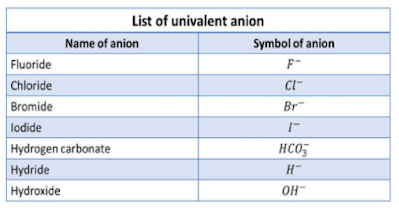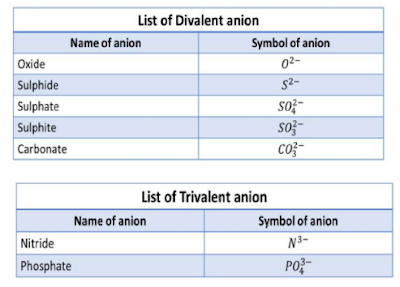⇒ aluminium has a +3 charge while chlorine has a -1 charge. The formation of aluminium chloride is shown below: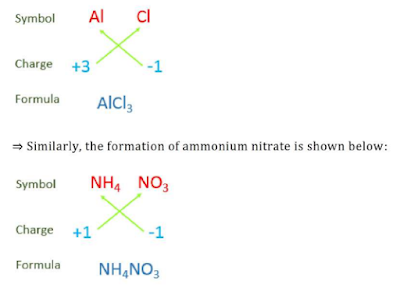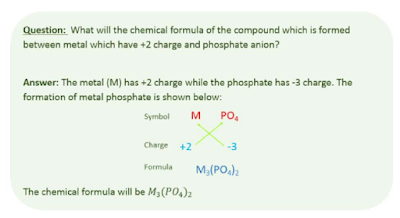Molecular Mass and Mole Concept

Molecular mass:

The molecular mass of a substance is the sum of the atomic masses of all the atoms in a molecule of the substance. For example, the molecular mass of water is calculated as shown below: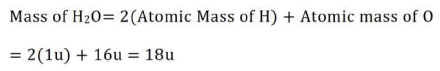Formula unit mass:

The formula unit mass of a substance is the sum of the atomic masses of all atoms in a formula unit of a compound. It is commonly used for ionic

compounds. For example, sodium chloride is an ionic compound whose mass is calculated as shown below: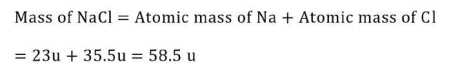Mole concept:

A mole is commonly used to describe a collection of particles that is, atoms, molecules, or ions.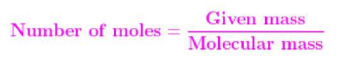⇒ When the atomic mass is expressed in grams, it is called Gram atomic mass. Similarly, when molecular mass is expressed in grams, it is called gram molecular mass.

⇒ The number of particles (atoms, molecules, or ions) present in 1 mole of any substance is fixed, with a value called the Avogadro Constant or Avogadro Number (NA). This number was named after the famous scientist, Amedeo Avogadro. The value of the Avogadro Constant is 6.023x1023.